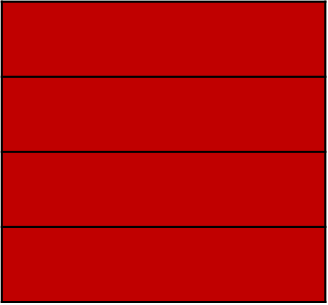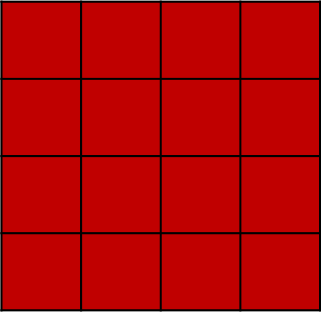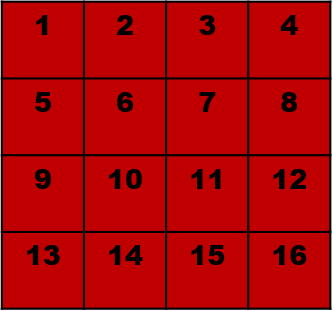1
visibility

Partition the given rectangle into 4 equal rows and 4 equal columns of same-sized squares, and then fill in the given blank.Number of small squares obtained =The correct answer is 16.

Solution:

Given the rectangle below:First, we divide the rectangle into 4 equal rows.Then, we divide this into 4 equal columns.Dividing the rectangle into 4 equal rows and 4 equal columns, results in small squares of the same size.

Next, we count these squares.So, the number of small squares made is 16.